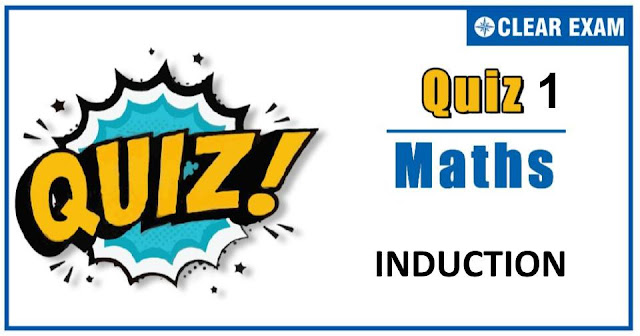## MATHEMATICS INDUCTION QUIZ-1Dear Readers,As per analysis for previous years, it has been observed that students preparing for JEE MAINS find Mathematics out of all the sections to be complex to handle and the majority of them are not able to comprehend the reason behind it. This problem arises especially because these aspirants appearing for the examination are more inclined to have a keen interest in Mathematics due to their ENGINEERING background. Furthermore, sections such as Mathematics are dominantly based on theories, laws, numerical in comparison to a section of Engineering which is more of fact-based, Physics, and includes substantial explanations. By using the table given below, you easily and directly access to the topics and respective links of MCQs. Moreover, to make learning smooth and efficient, all the questions come with their supportive solutions to make utilization of time even more productive. Students will be covered for all their studies as the topics are available from basics to even the most advanced.

Q1. Using mathematical induction, then numbers an's are defined by a0=1, a(n+1)=3n2+n+an,(n≥0) Then, an is equal to
•  n3+n2+1
•  n3-n2+1
•  n3-n2
•  n3+n2
Solution

Q2.For all n∈N,4n-3n-1 is divisible by
•  3
•  8
•  9
•  11
Solution
It can be checked that 4n-3n-1 is divisible by 9 for n=1,2,3,…

Q4. Let P(n):n2+n+1 is an even integer. If P(k) is assumed true ⇒P(k+1) is true. Therefore, P(n) is true
•  For n>1
•  For all n∈N
•  For n>2
•  None of these
Solution

Q5.For all n∈N,10n+3.4(n+2)+5 is divisible by
•  23
•  3
•  9
•  207

Q6. If (n):1+3+5+...+(2n-1)=n2 is
•  True for all n∈N
•  True for n>1
•  True for no n
•  None of these
Solution

Q8.For all n∈N,n3+2n is divisible by
•  3
•  8
•  9
•  11
Solution
For n=1,2,3, we find that n3+2n takes values 3,12 and 33, which are divisible by 3

Q9.x(x(n-1)-nα(n-1) )+αn (n-1) is divisible by (x-α)2 for
•  n>1
•  n>2
•  All n∈N
•  None of these
Solution

Q10. For all n∈N , 2∙4(2n+1)+3(3n+1) is divisible by
•  2
•  9
•  3
•  11
Solution#### Written by: AUTHORNAME

AUTHORDESCRIPTION## Want to know more

Please fill in the details below:

## Latest NEET Articles\$type=three\$c=3\$author=hide\$comment=hide\$rm=hide\$date=hide\$snippet=hide

Name

ltr
item
BEST NEET COACHING CENTER | BEST IIT JEE COACHING INSTITUTE | BEST NEET & IIT JEE COACHING: INDUCTION-QUIZ-1
INDUCTION-QUIZ-1